# Simple interest And Compound interest for IBPS , SBI , RBI | Quantitative Aptitude | Quiz-3

## Simple interest And Compound interest for IBPS , SBI , RBI

Simple interest And Compound interest plays a significant role in Quantitative Aptitude Section of banking exams such as IBPS, SBI and RBI PO and Clerk. You will get at least 4-5 questions from Simple interest And Compound interest in one of IBPS, SBI and RBI PO & clerk exam. So, aspirants should focus on Simple interest And Compound interest questions in detail. Here, we are providing you with the Simple interest And Compound interest questions quiz with the detailed solution so that you can easily prepare for Simple interest And Compound interest questions. We are providing here all-important latest pattern-based questions and Previous Year Questions of Simple interest And Compound interest of various Government Exam like IBPS, SBI, and RBI PO and Clerk exam. This Simple interest And Compound interest quiz we are providing is free. Attempt this Simple interest And Compound interest quiz to practice important questions with answers and solutions. And score better in IBPS, SBI and RBI PO and Clerk exam.

Simple interest And Compound interest Quiz to improve your Quantitative Aptitude for SBI Po & SBI clerk exam IBPS PO Reasoning , IBPS Clerk Reasoning , IBPS RRB Reasoning, LIC AAO ,LIC Assistant  and other competitive exam

1. A certain amount of money increases to Rs. 3,000 in 3 years and Rs. 3,500 in 4 years. Find the amount and the rate of interest.

(a)2500, 18%

(b) 3000, 12%

(c) 2000, 16.66%

(d) 2500, 14.87%

(e) 3200, 18%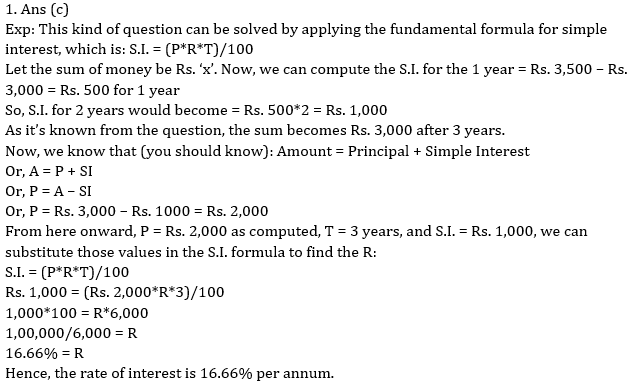2. A man borrows Rs 3000 at 10% compound interest per annum. At the end of each year he pays back Rs 1000. How much amount he pay at the end of 3rd year to clear all his dues?

(a) Rs 1683

(b) Rs 1530

(c) Rs 1453

(d) Rs 1430

(e) None of these3. Mr. Abhinav invested Rs. 20,000 with rate of interest at 20 p.c.p.a. The interest was compounded half yearly for first year and in the next year it was compounded yearly. What will be the total interest earned at the end of two years?

(a) Rs. 8,800

(b) Rs. 9,040

(c) Rs. 8,040

(d) Rs. 9,800

(e) None of these4. The difference between the S.I. on a certain amount at the rate of 10% per annum and compound interest which is compounded every 6 months for 2 years is Rs. 124.05. What is the principle ?

(a) Rs. 6,000

(b) Rs. 8,000

(c) Rs. 12,000

(d) Rs. 8,500

(e) None of these5. The difference between simple and compound interest on Rs. 6000 for 1 year at 20% per annum reckoned half yearly is:

(a) 120

(b) 60

(c) 180

(d) 72

(e) None of these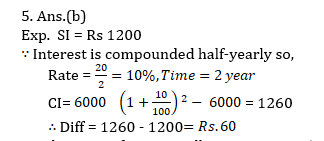6. What Sum of money will amount to Rs. 520 in 5 years and Rs. 568 in 7 years at simple interest?

(a)Rs 300

(b)Rs 350

(c)Rs  400

(d)Rs 450

(e)None of these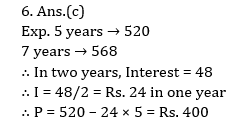7. If the rate of interest is 10% p.a. at which Rs. 12000 lent at the compound interest, half yearly, then what is the equivalent simple rate of interest for first year?

(a) 10.25%

(b) 9.24%

(c) 6.26%

(d) 8.42%

(e) None of these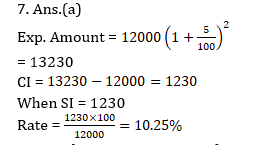8. CI and SI on a certain sum for 2 years at certain rate of interest are Rs. 220 and Rs. 200. What is the principal ?

(a) Rs. 2200

(b) Rs. 200

(c) Rs. 500

(d) Rs. 2000

(e) None of these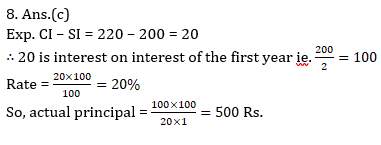9. A gave some amount at simple rate of interest of 10% to B for 3 years and B gave this amount to C at the rate of 20% for 3 years. If the profit of B was Rs. 1560 after 3 years, what amount A had given to B ?

(a) Rs. 6200

(b) Rs. 5400

(c) Rs. 5200

(d) Rs. 5600

(e) None of thee10. The difference between C.I. and S.I. on a certain sum at the rate of 10% per annum for 2 years is Rs. 122. Find that sum.

(a) 12,000

(b) 12,400

(c) 12,200

(d) 13,400

(e) None of these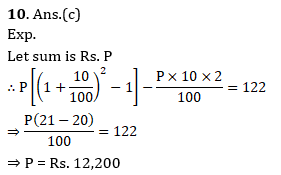### Online Mock Test Available on App as well as Web:### How to Access on App:-

1. Go to Playstore search Ambitious Baba or Click here to Install App

### You Can Read This Also:

ambitiousbaba.com need your support to Grow

I challenge you will get Best Content in Our PDFs with Detail solutions and Latest Pattern

Memory Based Puzzle E-book | 2016-19 Exams Covered

Get PDF here

Caselet Data Interpretation 200 Questions

Get PDF here
Puzzle & Seating Arrangement E-Book for BANK PO MAINS (Vol-1)

Get PDF here

### ARITHMETIC DATA INTERPRETATION 2020 E-book

Get PDF here
The Banking Awareness 500 MCQs E-book| Bilingual (Hindi + English)

Get PDF here

High Level DATA INTERPRETATION Practice E-BOOK

Get PDF her